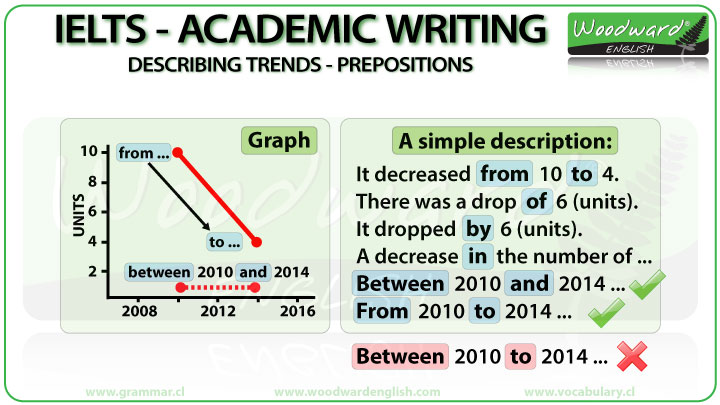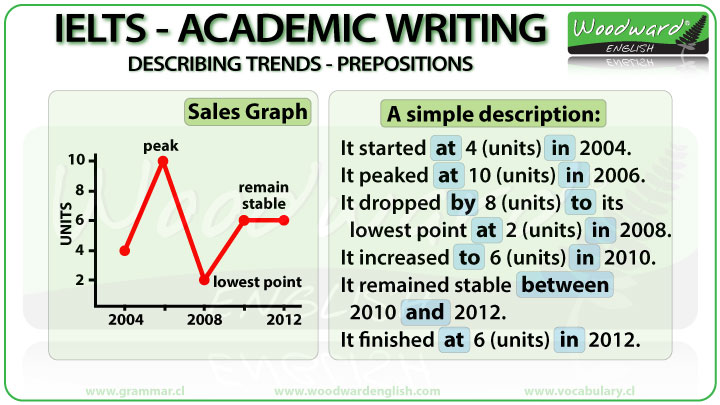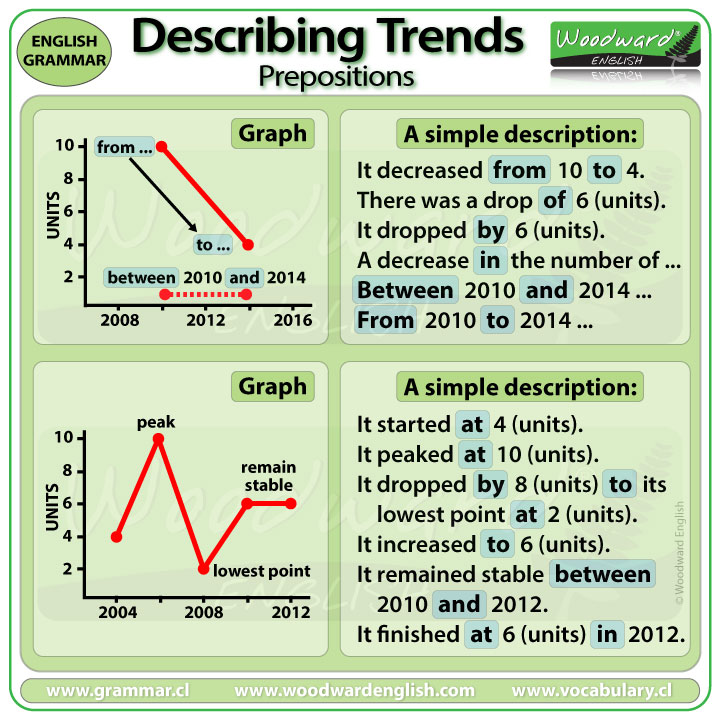Select Page

# IELTS Writing Task 1 – Describing Trends – Prepositions

### Prepositions – IELTS Academic Writing Task 1 Graphs – Describing Trends

Being able to use prepositions correctly is an important part of Writing Task 1 of the Academic version of the IELTS exam.

In this lesson we are going to learn when to use AT, ON, IN, BY, TO, and other prepositions correctly.

Let’s look at the words increase and decrease. They can be used as a noun or a verb.

It is important to know the difference of whether it is a noun or a verb because the preposition that comes after it will be different. For example:

### Increase and Decrease as Nouns

As nouns, increase, decrease, rise and fall normally have the preposition IN after them ….. when we do NOT specify a number.

• There was an increase in the number of emergency kits sold in 2012.
• There was a rise in sales after appearing on television.
• There has been a decrease in the consumption of sugar this decade.

Here I don’t specify any amount, or quantity, or numbers associated with the increase or decrease.

The moment we include a number or specify a number, we use the preposition OF after the noun increase or decrease.

• There was an increase of 70% in sales of emergency kits sold in 2012.

We use OF because there is a specific number after it… 70%

In this example you could also change the order a little.

• There was a 70% increase in sales of emergency kits sold in 2012.

Notice how the percentage number goes before the noun increase and we now use the preposition IN because there is no specific number after it.

In summary:

INCREASE (noun) + IN  … when we do NOT specify a number
INCREASE (noun) + OF… when we specify a number

Two example sentences:

• There has been an increase in the number of students here this semester.
• There has been an increase of 500 students here this semester.

We use IN in the first sentence because we do NOT specify a number.
We use OF in the second sentence because we specify a number, in this case 500.

### Increase and Decrease as Verbs

So, we have seen which prepositions to use after increase and decrease when they are nouns, but what do we use when they are verbs?

When increase and decrease are verbs, they normally have the following prepositions after them:

Increase FROM X (the first amount) TO Y (the final amount).

• Sales increased from 100 to 130 units.

FROM is with the start point of a trend.
TO is with the end point of a trend

What is the difference between 100 and 130? It is 30.

To show this difference we can use the preposition BY.

Increase (or decrease etc.) + BY + the amount of change.

• Sales increased by 30 units.

BY = the amount of change between two different periods of time or values.

When we use a percentage instead of another number, the preposition BY is optional.

• Sales increased by 30%. … OR
• Sales increased 30%. (both are correct)

(Both example sentences above are correct)

### BY vs. TO

Let’s look at the following sentences:

• It declined by 5%
• It declined to 5%

We have a different preposition in each sentence and they both change the meaning of it significantly. Let’s see how…

What is the difference between these two prepositions if the starting point is 100%?
It declined BY 5% means it only drops a small amount from 100% to 95%

BY is used to show the difference between the two numbers.
The difference between 100 and 95 which is 5.

It declined TO 5% means it fell from 100% all the way down to 5% which is a huge drop.
We use TO because we are talking about the end point, in this case the end point is 5%.

It declined to 5% is a short way of saying: It declined from 100% to 5%.

Sometimes we don’t use the FROM part as that number has already been mentioned in a previous part and we just use the Preposition TO to mention the end point.

### Prepositions with Time Periods

When we talk about specific months or years, we use the preposition IN.

IN + Month
IN + Year
IN + the (first, second, etc.) QUARTER
(A quarter is a period of 3 months of the year)

Example sentences:

• 500 units were sold in December.
• The number of accidents doubled in 20-17.
• Profits increased in the second quarter.

We use BETWEEN X AND Y when we want to show a range of time, which means it has a start time and an end time.

• Crude Oil prices rose sharply between 1973 and 1980.

We can use SINCE when we have a starting point and the trend continues until now. SINCE is mostly used with a perfect tense.

• Property prices in Auckland have increased significantly since 2011.

We use SINCE because the prices continue to increase until today.

See our lesson about the difference between FOR and SINCE.

### Starting and Ending – Prepositions

After verbs that start and end, we mostly use the preposition AT before a number:

To start AT
To begin AT
To end AT
To finish AT

Some example sentences:

• Initial prices started at \$300 per unit.
• Production began at 70 units per day in March.
• Production ended at 90 units per day in August.

We can also use the preposition AT with the verb To Peak

• Crime peaked at 200 incidents per night in April.

When the trend remains constant or steady, we can also use the preposition AT:

• Over the last decade, the population of the town has remained steady at around 10,000 inhabitants

Notice how in the first part we said: over the last decade.

We use OVER and DURING with a time period

• Sales increased over the next three months. … is the same as …
• Sales increased during the next three months.

### Summary Graph

Let’s look at this simple graph to apply many of the prepositions we have talked about.

What is the direction of this trend?It goes down. So to describe this trend we could use a word like decrease or drop or decline.

For now we will say… it decreased. It could be sales decreased, production decreased, it could be many things. At the moment I’m interested in the prepositions that come next.

What is the starting point? 10 units.
What is the end point? 4 units.

So we could say:

• It decreased from 10 to 4 units.

FROM is used with the starting point, in this case 10,
TO is used with the end point, in this case 4.

Now, what is the difference between 10 and 4. It is a difference of 6 so we could say:
There was a drop, or decrease, or decline, OF 6 units.

• There was a drop of 6 units.

We use OF after a noun, in this case the noun drop.

How about if we use the verb form of drop. We would say:

• It dropped by 6 units.

Notice how we use OF after the noun form of drop, and BY after the verb form of drop when showing the change or difference between two numbers.

• There was a drop of 6 units.
• It dropped by 6 units.

But if we do not include a specific number, we use the preposition IN after the noun.

• There was a decrease in the number of X. … OR
• There was a drop in the number of X.

Here we haven’t specified a number.
We don’t know if it 6 or 6 million. We just know there was a drop or decline.

Now compare the two together:

• There was a drop of 6 units.
• There was a drop in the number of…

The preposition changes because the first sentence has a specific number while the second sentence doesn’t give us a specific number so we say “the number”.

• OF + specific number …
• IN + the number of …

… and then sales, units, or whatever

If we look at the chart again, what is the time period that the decrease happens?

The decrease happens from one date to another date. Those two dates, or years, are 2010 and 2014. Even though those numbers don’t appear written on the graph, we can see the time intervals in the bottom axis are divided into two-year periods.

For time periods that include two dates, we can say:

• Between 2010 and 2014
• From 2010 to 2014

But we cannot mix the prepositions and say:
Between 2010 to 2014 … NO this is incorrect.

Let’s look at another simple graph:When does this graph start?

• It starts in 2004.

We use IN because we are talking about something that happened in a specific year… in 2004.

And what happened then?
Something started. Let’s imagine it’s talking about sales of computer units.

How many units were sold in 2004?
4 units were sold.

So what preposition is used as a part of the simple description?

• It started at 4 units in 2004.

We use start + AT + the number.

Then what happened? Sales increased.

They increased TO its highest point.

This highest point is called a peak. Peak can be a noun or a verb. Let’s use the verb:

• It peaked at 10 units.

We use the preposition AT after the verb PEAK.

We could also add the time the peak happened… in 2006.

Of course if we say:

• It started at 4 units in 2004. It peaked at 10 units in 2006.

This sounds repetitive. We are just doing these simple descriptions to learn the correct prepositions.

Just so you know, instead of saying: It peaked at 10 units in 2006.
We could say: It peaked at 10 units two years later.

That shows the examiner you can vary your vocabulary.

Then what happened after it peaked?
We can see that sales went down. Sales dropped.

How much did it drop?

• It dropped by 8 units.

Why do we use BY in this case?

Because it is the difference between the starting point, 10 units, and the end point, 2 units. The difference between 10 and 2 is… 8, so 8 units.

To show this difference we use the preposition BY. It dropped by 8 units.

We could also say it dropped TO two units though saying “to two” together sounds strange.

The sales of only two units that year was the lowest amount that was sold, which makes it its lowest point.

• Sales dropped to its lowest point.

So bringing it all together we say:

• It dropped by 8 units to its lowest point.

If we want to add the number that is at its lowest point, which is a good thing to do in order to give a more complete response, then we use the preposition AT after lowest point, in this case it dropped to its lowest point AT 2 units.

You see we also added IN + the year to state when this lowest point happened, IN 2008. So the complete sentence is:

• It dropped by 8 units to its lowest point at 2 units in 2008.

Then what happened?
We can see the trend going up.
Sales increased again, this time TO 6 units.

• It increased to 6 units sold in 2010.

We use the preposition TO after the verb INCREASE when referring to increasing to a number.

Then we can see it didn’t make a change up or down. It remained stable or constant.

What is the time period that it remained stable? We have 2010 and 2012. We can say:

• Sales remained stable between 2010 and 2012.

See how we used the prepositions BETWEEN x AND y …

Another alternative is to use the prepositions …. FROM x TO y.

• Sales remained stable from 2010 to 2012.

Just remember we cannot mix the prepositions.
BETWEEN is always with AND.
FROM is always with TO.

So, where does the graph finish?

• It finished at 6 units in 2012

We use AT with the verbs finish and end. Just like you start at … you finish at.

• It finished at 6 units sold in 2012.This was a simple overview of common prepositions you will be using in Writing Task 1 of the Academic Version of the IELTS exam. I recommend seeing our other lessons about prepositions in general.

Lesson tags: Academic, Describing Trends, IELTS, Prepositions, Writing
Back to: IELTS Preparation Course > IELTS Writing